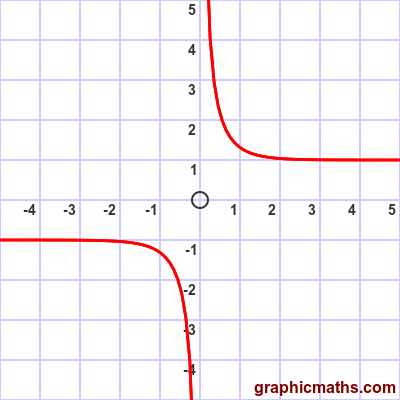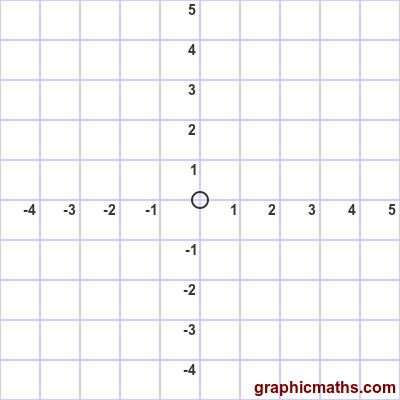# coth function

By Martin McBride, 2021-02-06
Tags: coth tanh inverse hyperbolic function
Categories: hyperbolic functions pure mathematics

The coth function is a hyperbolic function. It is also known as the hyperbolic cotangent function.

Here is a video that explains the cosech, sech, and coth functions:

## Equation and graph

The coth function is the reciprocal of the tanh function, and is only defined for non-zero values:

$$\operatorname{coth}{x} = \frac{1}{tanh{x}} = \frac{\cosh{x}}{\sinh{x}}$$

(This is analogous with trig functions, where cot is the reciprocal of tan.)

Substituting the formula for tanh gives:

$$\operatorname{coth}{x} = \frac{e^{x}+e^{-x}}{e^{x}-e^{-x}}$$

Here is a graph of the function:## coth as cosh divided by sinh

The coth function involves two functions, $\cosh{x}$ divided by $\sinh{x}$. This animation illustrates this:When $x$ is large and negative, $\sinh{x}$ becomes ever closer to $-\cosh{x}$. The value of $\coth{x}$ therefore tends towards -1.

When $x$ is large and positive, $\sinh{x}$ becomes ever closer to $\cosh{x}$. The value of $\coth{x}$ therefore tends towards 1.

As $x$ approaches zero from the negative direction, $\sinh{x}$ approaches zero, so $\coth{x}$ tends towards -∞.

As $x$ approaches zero from the positive direction, $\sinh{x}$ approaches zero, so $\coth{x}$ tends towards +∞.

## Other forms of the equation

If we take the two alternative forms of the tanh function, and invert them, we get two alternative forms of the coth function:

$$\operatorname{coth} = \frac{e^{2x}+1}{e^{2x}-1}$$

$$\tanh{x} = \frac{1+e^{-2x}}{1-e^{-2x}}$$